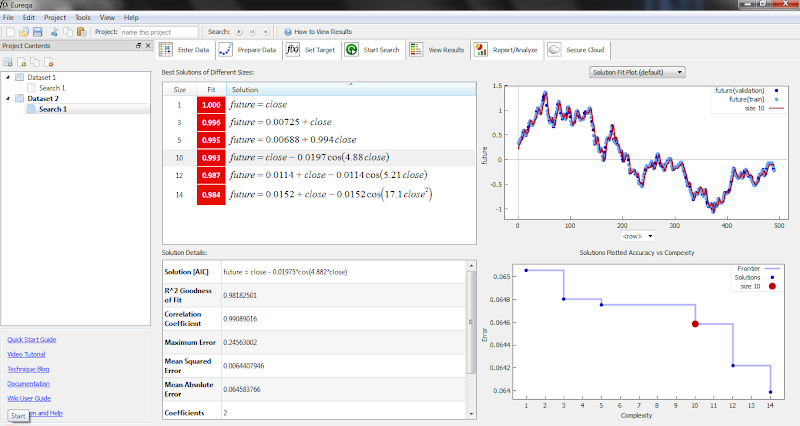## Eureka: genetic programming software

By JohnLast 2738 days ago Comments (5)
http://creativemachines.cornell.edu/eureqa

Eureqa (pronounced "eureka") is a software tool for detecting equations and hidden mathematical relationships in your data. Its goal is to identify the simplest mathematical formulas which could describe the underlying mechanisms that produced the data. Eureqa is free to download and use.

This software essentially does the same job as the commercial chaos hunter application however the commercial application also transfers the generated mathematical formulas to the trading plateforms.

Here you need to figure out how to do that on your own.

Anyway the simplest thing that can be done is to model the current trend for example.

Here I  would suggest for reading two interesting articles:

What will cause the Singularity? : this is very interesting article it appears that Eureka is an oustanding genetic programming software: “That’s a pretty big deal in the world of evolutionary algorithms”

Product review of Chaos hunter from traders.com : this a basic article about the practical use of the genetic programming software in trading strategies: two methods the definition of a treshold or boolean strategy.

Let me summarize in a simple way the neural nets do the same job as the genetic programming however the Neural nets will store their solution as a data set of weights that has no meaning for a human. The genetic software forxe that the solution is described by mathematical formulas that a human can understand. And that is why so much hype is there in the scientific community.

• JohnLast 2737 days ago

This comes as a midnight joke but take a look. I installed eureka then loaded daily data of EURUSD, then I put as prediction target the next 2 days close. Then started and finally the software told me that one of the best predictions for the next two days is the today close for daily frame. After so much data mining this obvious thing came as an uppercut.

LOL.От Fundamental

• jaguar1637 2737 days ago

ok, it's a regression algorithm (levenmark ?)  or a neuronal system that brought those results ?

• JohnLast 2737 days ago

This is genetic programming, the algorythm evolve basic equations in order to achieve the best fit. As a result we havr equations that we can understand and not weights.

In that way you can understand the results. For example if you do the same thing with a neural net you will get weigts and if you run the model you will get predictions but you will never know the idea behind even if basically the prediction is the current close ;).

• JohnLast 2737 days ago

I think this tool eureka is great in order to find relationships between the forex market and the fundamental data. In fact this tool was built with that idea to help scientifists to generate formulas helping them to understand complex relationships.

Another possible use would be to try it for statistical arbitrage, not necesseraly in Forex.

• JohnLast 2736 days ago

I think that it will be possible to use the formula generated by Eureka and with them to modify the output in the indicator and by that to get a predictive indicator without much work.

In the example from the screen shot we have several formulas:

We take the indicator output then we implement the formula directly in metatrader.

For example: future = 0.00725 + close

we take the output from the indicator and we add 0.00725.

It is interesting to note that future=close is a prediction by itself.

So.

1. We use an indicator and we calculate it in MT4

2. Then we export the data

3. We read the data in Eureka

4. We do calculations

5. We implement the formula

The key is to know what to predict in the example above I tried to predict the next 3 days of close using daily data and I got future = close ;).